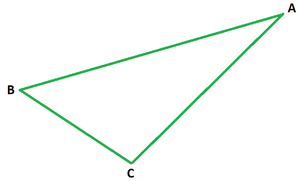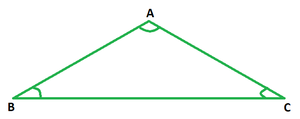Open In App

# How to find the area and perimeter of an equilateral triangle?

In mathematics or in daily lives geometry and geometrical shapes always play an essential role. Starting from a simple tray to a big building, we are always surrounded by geometry in different shapes and sizes. The shape and surface to be taken up by such shapes need to be calculated so as to store them without any difficulty, or to meet the needs of the people using them. Such study or computation is given a special name in the field of mathematics.

What is Mensuration?

Whenever the dimensions of various geometrical shapes are computed and their area or capacity is measured in mathematics, it is termed mensuration. Mensuration also helps to calculate the dimensions of other shapes from their surface area and capacity.

Triangle

Such a 2-D shape is formed by joining three points, two of which are collinear is called a triangle. As the name suggests, such a shape has exactly three angles, the sum of whom is always 180°. ‘Tri’ suggests three vertices, sides, angles of this enclosed shape. It is geometrically expressed as ‘△’. In the figure below, ABC is a triangle with points B and C being collinear. Clearly, △ABC has three sides AB, BC, and CA, and three angles ∠ABC, ∠BCA, and ∠CAB.### Types of Triangles

Four main types of triangles are as follows:

• Scalene Triangle: Such a triangle that has angles that vary in measure and all three sides varying in length is called a scalene triangle.
• Isosceles Triangle: Such a triangle that has two sides that are equal in length is called an isosceles triangle.
• Equilateral Triangle: Such a triangle whose all sides are equal in length and all angles are equal in measure as well is called an equilateral triangle.
• Right Triangle: Such a triangle that has an angle that measures 90° is called a right triangle. Such a triangle has three sides, out of which the hypotenuse is the longest and is opposite the 90 degrees angle.

### Equilateral Triangle

Such a triangle whose all sides are equal in length and all angles are equal in measure as well is called an equilateral triangle. Since all the angles of a triangle total 180 degrees, hence each angle of an equilateral triangle shall measure 60°. In the figure below △ABC is an equilateral triangle whose three sides are equal in length, i.e., Ab = BC = CA, and each angle measures 60°, i.e., ∠ABC = ∠BCA = ∠CAB = 60°.Area of an equilateral triangle

• Given the length of the side of an equilateral triangle, its area can be calculated using the following formula:

Area of equilateral triangle =• Given the length of altitude as well as the side of an equilateral triangle, its area can be calculated using the following formula:

Area of equilateral triangle = (1/2) × Side × Altitude

Perimeter of an equilateral triangle

The perimeter of a geometrical shape is defined as the sum of the lengths of all sides of the said shape. Hence, in order to calculate the perimeter of any triangle, be it scalene or isosceles or equilateral, one needs to know the lengths of sides of the said triangle to add them up to get the desired result.

Since an equilateral triangle is the one whose all sides are equal in length, the sum of all the three (equal) sides would result in the sum being three times the length of an individual side of the given triangle. Hence, if the length of one side of an equilateral triangle is ‘a’ units:

Perimeter = a + a + a = 3a units, thrice the length of one side.

### Sample Questions

Question 1. Find the side of an equilateral triangle, given its perimeter is 69 cm.

Solution:

We know perimeter of an equilateral triangle = 3a

⇒ 3a = 69 cm

⇒ a = 69/ 3 cm = 21 cm

Hence, the length of side of the triangle is 21 cm.

Question 2. Find the length of the side of the equilateral triangle whose area is 60 cm2 and altitude is 15 cm.

Solution:

We know area of an equilateral triangle = (1/2) × Side × Altitude

⇒ 60 = (1/2) × Side × 15

⇒ 4 = (1/2) × Side

⇒ Side = 4 × 2

⇒ Side = 8 cm

Question 3. Find the area of an equilateral triangle whose perimeter is 24 cm.

Solution:

We know perimeter of an equilateral triangle = 3a

⇒ 3a = 24 cm

⇒ a = 24/ 3 cm = 8 cm

Area =Area = 16√3 cm2

Question 4. Find the area and perimeter of an equilateral triangle whose side is 9 cm.

Solution:

Area = \frac{\sqrt3}{4}×a×a = \frac{\sqrt3}{4}×9×9 = 81√3/ 4 cm2.

Perimeter = 3a = 3(9) cm = 27 cm.